# Exam-Style Questions on Algebra

## Problems on Algebra adapted from questions set in previous Mathematics exams.

### 1.

GCSE Higher

Solve the following linear equations showing clearly each step of your working.

(a) $$\frac{6}{x} = 24$$.

(b) $$11x - 5 = 9(x + 9)$$.

### 2.

GCSE Higher

The diagram shows an isosceles triangle (not drawn to scale).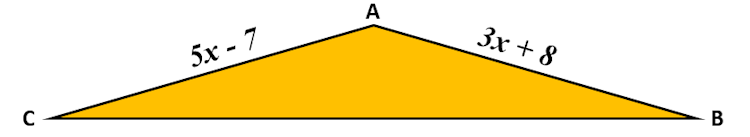Angle ABC = angle ACB, AB = $$3x+8$$ and AC = $$5x-7$$.

Use an algebraic method to find the value of $$x$$.

### 3.

GCSE Foundation

The diagrams show a triangle and a rectangle (not drawn to scale).

The area of the rectangle is six times larger than the area of the triangle. Find the value of $$x$$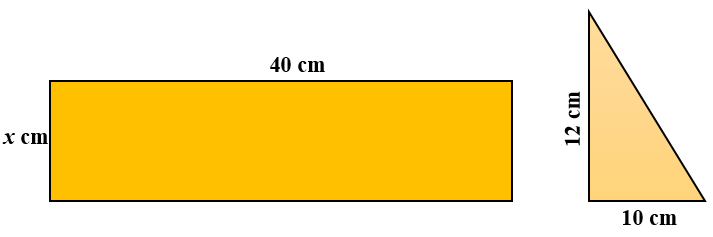### 4.

GCSE Higher

The perimeter of the triangle is the same length as the perimeter of the square.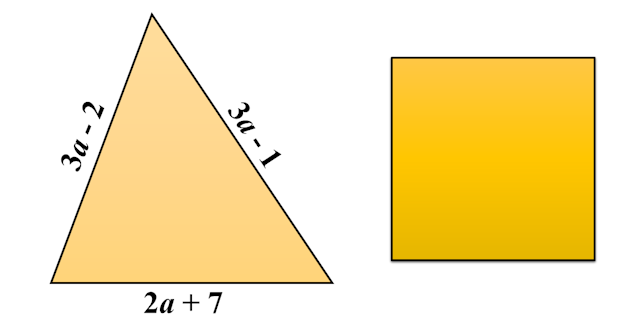Find an expression for the length of one side of the square in terms of a. Give your answer in its simplest form.

### 5.

GCSE Higher

Multiply out and simplify:

$$(x-6)^2$$

### 6.

GCSE Higher

Aimee, Natasha and Ruby play hockey.

• Aimee has scored 6 more goals than Natasha.
• Ruby has scored 5 more goals than Aimee.

If altogether they have scored 35 goals, how many goals did they each score?

### 7.

GCSE Higher

Luke, Leia and Han swim lengths of a swimming pool to raise money for charity.• Luke swims 20 more lengths than Han
• Leia swims twice as many lengths as Han
• Altogether they swim 232 lengths.

How many lengths did each person swim?

### 8.

GCSE Higher

Simplify then find the square root of this expression:

$$\frac{y}{(1-y)^2} - \frac{y}{1-y}$$

### 9.

GCSE Higher

Two rectangles, not drawn to scale, are shown below. All measurements are in centimetres. Both rectangles have the same areas. Work out the perimeter of the rectangle on the left.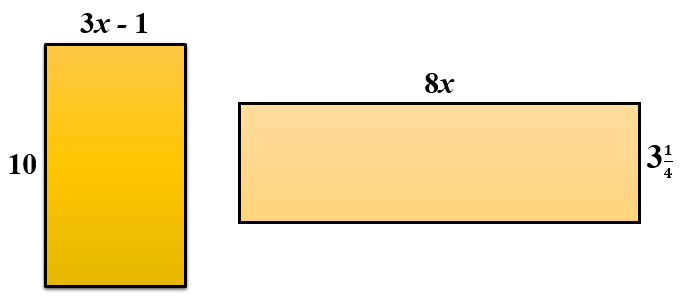### 10.

GCSE Higher

The area of rectangle PQRS (not to scale) is 80cm2.

(a) Show that $$x^2 + 14x = 40$$.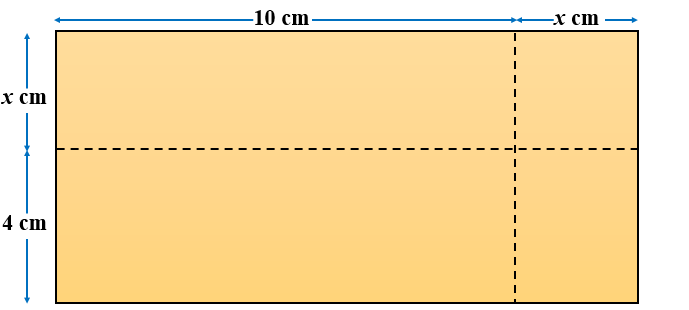(b) Find the value of $$x$$ giving your answer correct to three significant figures.

### 11.

GCSE Higher

Expand and simplify the following expression:

$$(2x^2 + 3)(4x - 5) - 8x(x^2 - 7)$$

### 12.

GCSE Higher

Show that you understand equations and inequalities by answering the following:

(a) Solve $$5x^2=80$$

(b) Solve $$8x + 2 \gt x + 7$$

(c) Write down the largest integer that satisfies $$8x - 2 \lt 25$$

(d) Solve the following pair of equations

$$3x + 5y = 21$$ $$8x - 5y = 1$$

### 13.

IGCSE Extended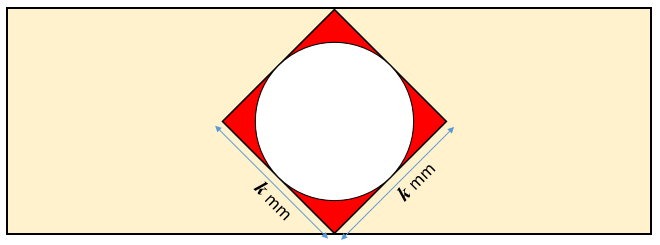A circle is drawn inside a square so that it touches all four sides of the square.

(a) If the sides of the square are each $$k$$ mm in length and the area of the red shaded region is $$A$$ mm2 show that:

$$4A=4k^2-\pi k^2$$

(b) Make $$k$$ the subject of the formula $$4A=4k^2-\pi k^2$$

### 14.

GCSE Higher

One is added to the product of two consecutive positive even numbers. Show that the result is a square number.

### 15.

GCSE Higher

(a) Simplify the following expression.

$$\frac{x^2 - 4}{3x^2 + 13x + 14}$$

(b) Make b the subject of the following formula.

$$a = \frac{7(3b-c)}{b}$$

### 16.

GCSE Higher

Rätselzeit school has an annual sports day in which the mathematics teachers are in charge of the scoring system.

The points scored for throwing the discus are worked out using the formula:

$$p = 15(d – 4.2)$$

where $$p$$ is the number of points scored when the discus is thrown a distance of $$d$$ metres.

(a) How many points does Homer score for throwing the discus a distance of 52 metres?

(b) How far did Marge throw the discus if she scored 492 points?

The points scored for running 400 metres are worked out using the formula:

$$p = 6(95 – t)^2$$

where $$p$$ is the number of points scored when the time taken is $$t$$ seconds.

(c) Lisa scores 1014 points for the 400 metres race. Work out the time, in seconds, it took Lisa.

The formula for the number of points scored in the 400 metres race should not be used for $$t\gt n$$.

(d) State the value of $$n$$ giving a reason for your answer.

### 17.

GCSE Higher

Betsy thinks that $$(3x)^2$$ is always greater than or equal to $$3x$$.

Is she is correct?

Show your working to justify your decision

### 18.

GCSE Higher

Factorise the following expression

$$6x^2-x-15$$

### 19.

GCSE Higher

Given that:

$$x^2 : (5x + 3) = 1 : 3$$

find the possible values of $$x$$.

### 20.

GCSE Higher

The expression below can be written as a single fraction in the form $$\frac{a-bx}{x^2-25}$$ where $$a$$ and $$b$$ are integers.

$$\frac{x-4}{x-5} - 2 + \frac{x+1}{x+5}$$

Work out the value of $$a$$ and the value of $$b$$.

### 21.

GCSE Higher

If $$y = 5x^4 + 3x^2$$ and $$x=\sqrt{w+2}$$, find $$w$$ when $$y = 12$$ showing each step of your working.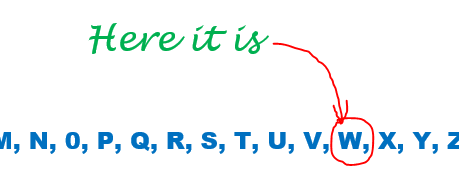If you would like space on the right of the question to write out the solution try this Thinning Feature. It will collapse the text into the left half of your screen but large diagrams will remain unchanged.

The exam-style questions appearing on this site are based on those set in previous examinations (or sample assessment papers for future examinations) by the major examination boards. The wording, diagrams and figures used in these questions have been changed from the originals so that students can have fresh, relevant problem solving practice even if they have previously worked through the related exam paper.

The solutions to the questions on this website are only available to those who have a Transum Subscription.

Exam-Style Questions Main Page

Search for exam-style questions containing a particular word or phrase:

To search the entire Transum website use the search box in the grey area below.

## Comments:For All:

©1997-2021 WWW.TRANSUM.ORG